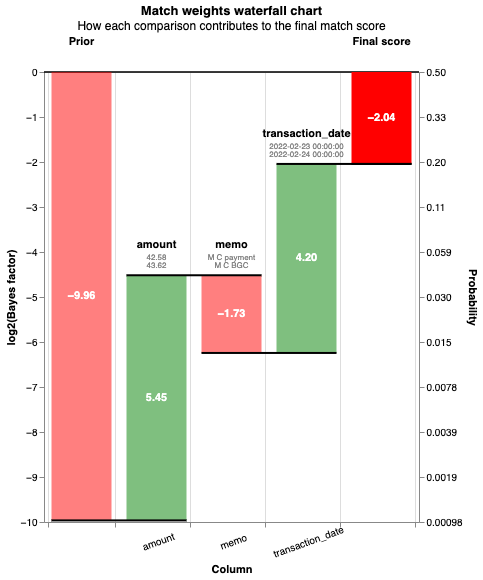This example shows how to perform a one-to-one link on banking transactions.

The data is fake data, and was generated has the following features:

• Money shows up in the destination account with some time delay
• The amount sent and the amount received are not always the same - there are hidden fees and foreign exchange effects
• The memo is sometimes truncated and content is sometimes missing

Since each origin payment should end up in the destination account, the probability_two_random_records_match of the model is known.

# Use arrow to read in data to ensure date types are correct
from pyarrow import parquet as pq
import altair as alt
alt.renderers.enable('mimetype')

df_origin = df_origin.slice(length=1_000)
df_destination = df_destination.slice(length=1_000)
f"There are {df_origin.num_rows:,.0f} records to match"

'There are 1,000 records to match'

# Some sample records

ground_truth memo transaction_date amount unique_id
0 0 MATTHIAS C paym 2022-03-28 36.36 0
1 1 M CORVINUS dona 2022-02-14 221.91 1

In the following chart, we can see this is a challenging dataset to link: - There are only 151 distinct transaction dates, with strong skew - Some 'memos' are used multiple times (up to 48 times) - There is strong skew in the 'amount' column, with 1,400 transactions of around 60.00

# Simple settings just for exploratory analysis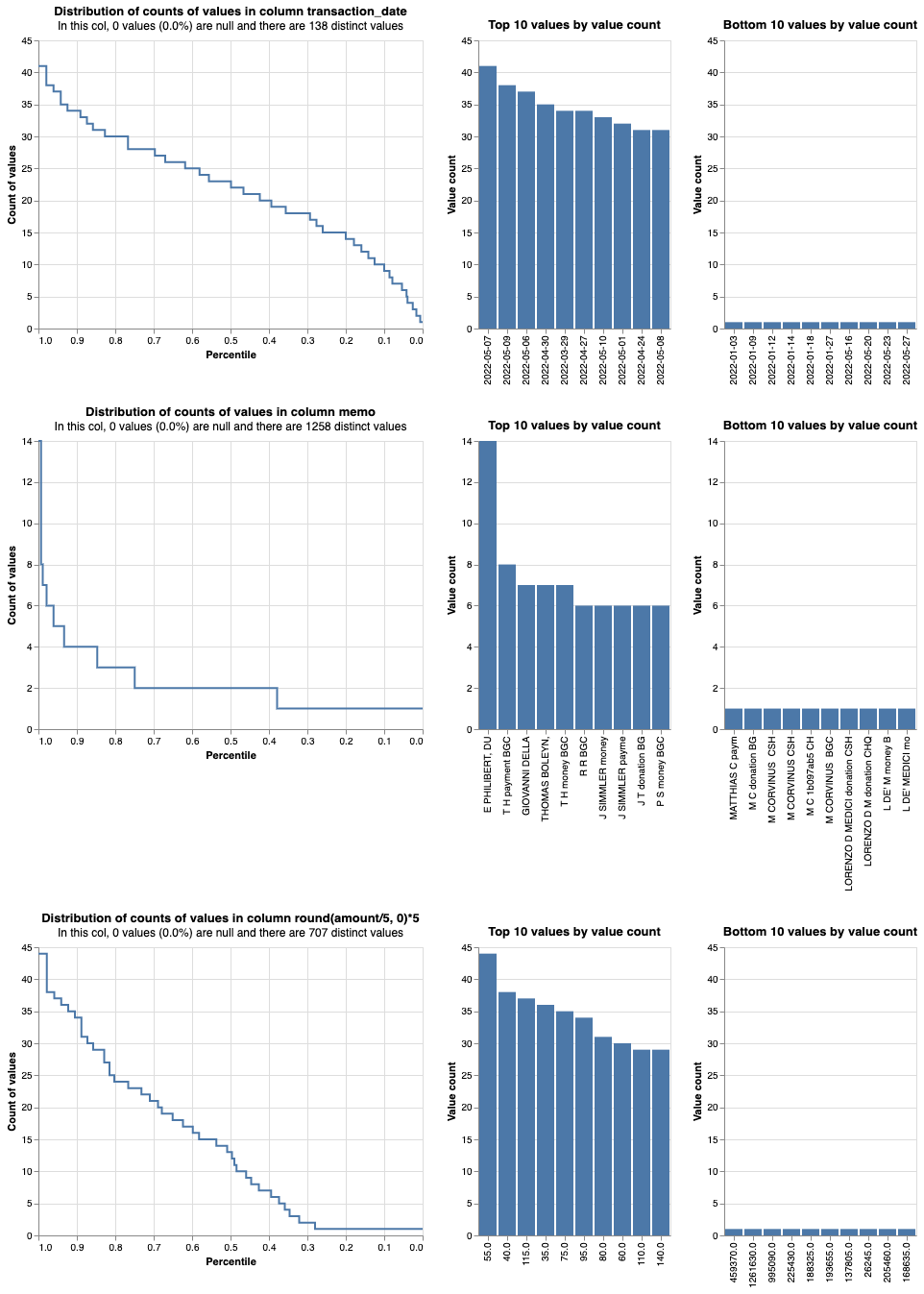# Design blocking rules that allow for differences in transaction date and amounts

blocking_rule_date_1 = """
strftime(l.transaction_date, '%Y%m') = strftime(r.transaction_date, '%Y%m')
and substr(l.memo, 1,3) = substr(r.memo,1,3)
and l.amount/r.amount > 0.7   and l.amount/r.amount < 1.3
"""

# Offset by half a month to ensure we capture case when the dates are e.g. 31st Jan and 1st Feb
blocking_rule_date_2 = """
strftime(l.transaction_date+15, '%Y%m') = strftime(r.transaction_date, '%Y%m')
and substr(l.memo, 1,3) = substr(r.memo,1,3)
and l.amount/r.amount > 0.7   and l.amount/r.amount < 1.3
"""

blocking_rule_memo = """
substr(l.memo,1,9) = substr(r.memo,1,9)
"""

blocking_rule_amount_1 = """
round(l.amount/2,0)*2 = round(r.amount/2,0)*2 and yearweek(r.transaction_date) = yearweek(l.transaction_date)
"""

blocking_rule_amount_2 = """
round(l.amount/2,0)*2 = round((r.amount+1)/2,0)*2 and yearweek(r.transaction_date) = yearweek(l.transaction_date + 4)
"""

blocking_rule_cheat = """
l.unique_id = r.unique_id
"""

[
blocking_rule_date_1,
blocking_rule_date_2,
blocking_rule_memo,
blocking_rule_amount_1,
blocking_rule_amount_2,
blocking_rule_cheat,
]
)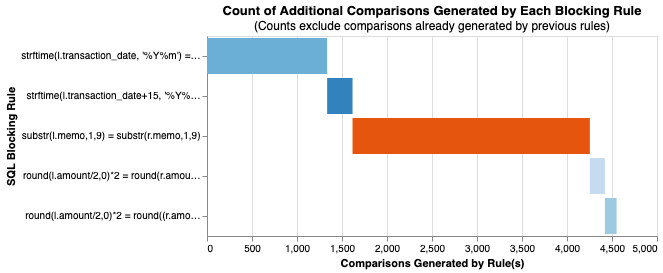# Full settings for linking model

comparison_amount = {
"output_column_name": "amount",
"comparison_levels": [
cl.null_level("amount"),
cl.exact_match_level("amount"),
cl.percentage_difference_level("amount",0.01),
cl.percentage_difference_level("amount",0.03),
cl.percentage_difference_level("amount",0.1),
cl.percentage_difference_level("amount",0.3),
cl.else_level()
],
"comparison_description": "Amount percentage difference",
}

within_n_days_template = "transaction_date_r - transaction_date_l <= {n} and transaction_date_r >= transaction_date_l"

comparison_date = {
"output_column_name": "transaction_date",
"comparison_levels": [
cl.null_level("transaction_date"),
{"sql_condition": within_n_days_template.format(n=1), "label_for_charts": "1 day"},
{"sql_condition": within_n_days_template.format(n=4), "label_for_charts": "<=4 days"},
{"sql_condition": within_n_days_template.format(n=10), "label_for_charts": "<=10 days"},
{"sql_condition": within_n_days_template.format(n=30), "label_for_charts": "<=30 days"},
cl.else_level()
],
"comparison_description": "Transaction date days apart",
}

from splink.duckdb import duckdb_comparison_library as cl

settings = {
"probability_two_random_records_match": 1 / len(df_origin),
"blocking_rules_to_generate_predictions": [
blocking_rule_date_1,
blocking_rule_date_2,
blocking_rule_memo,
blocking_rule_amount_1,
blocking_rule_amount_2,
blocking_rule_cheat
],
"comparisons": [
comparison_amount,
cl.jaccard_at_thresholds(
"memo", [0.9, 0.7]
),
comparison_date
],
"retain_intermediate_calculation_columns": True,
"retain_matching_columns": True,
}

linker = DuckDBLinker([df_origin, df_destination], settings,input_table_aliases=["__ori", "_dest"])

linker.estimate_u_using_random_sampling(target_rows=1e6)

----- Estimating u probabilities using random sampling -----

Estimated u probabilities using random sampling

Your model is not yet fully trained. Missing estimates for:
- amount (no m values are trained).
- memo (no m values are trained).
- transaction_date (no m values are trained).


linker.estimate_parameters_using_expectation_maximisation("l.memo = r.memo")


----- Starting EM training session -----

Estimating the m probabilities of the model by blocking on:
l.memo = r.memo

Parameter estimates will be made for the following comparison(s):
- amount
- transaction_date

Parameter estimates cannot be made for the following comparison(s) since they are used in the blocking rules:
- memo

Iteration 1: Largest change in params was -0.555 in the m_probability of amount, level Exact match
Iteration 2: Largest change in params was -0.213 in the m_probability of transaction_date, level 1 day
Iteration 3: Largest change in params was 0.00794 in probability_two_random_records_match
Iteration 4: Largest change in params was 0.000315 in the m_probability of transaction_date, level <=30 days
Iteration 5: Largest change in params was -0.000177 in the m_probability of amount, level All other comparisons
Iteration 6: Largest change in params was -0.000159 in the m_probability of amount, level All other comparisons
Iteration 7: Largest change in params was -0.000144 in the m_probability of amount, level All other comparisons
Iteration 8: Largest change in params was -0.000131 in the m_probability of amount, level All other comparisons
Iteration 9: Largest change in params was -0.000119 in the m_probability of amount, level All other comparisons
Iteration 10: Largest change in params was -0.000109 in the m_probability of amount, level All other comparisons
Iteration 11: Largest change in params was -9.97e-05 in the m_probability of amount, level All other comparisons

EM converged after 11 iterations

Your model is not yet fully trained. Missing estimates for:
- memo (no m values are trained).


<EMTrainingSession, blocking on l.memo = r.memo, deactivating comparisons memo>

session = linker.estimate_parameters_using_expectation_maximisation("l.amount = r.amount")


----- Starting EM training session -----

Estimating the m probabilities of the model by blocking on:
l.amount = r.amount

Parameter estimates will be made for the following comparison(s):
- memo
- transaction_date

Parameter estimates cannot be made for the following comparison(s) since they are used in the blocking rules:
- amount

Iteration 1: Largest change in params was 0.495 in probability_two_random_records_match
Iteration 2: Largest change in params was 0.134 in probability_two_random_records_match
Iteration 3: Largest change in params was 0.139 in probability_two_random_records_match
Iteration 4: Largest change in params was 0.0293 in probability_two_random_records_match
Iteration 5: Largest change in params was 0.00393 in probability_two_random_records_match
Iteration 6: Largest change in params was 0.000522 in probability_two_random_records_match
Iteration 7: Largest change in params was 6.93e-05 in probability_two_random_records_match

EM converged after 7 iterations

Your model is fully trained. All comparisons have at least one estimate for their m and u values


linker.match_weights_chart()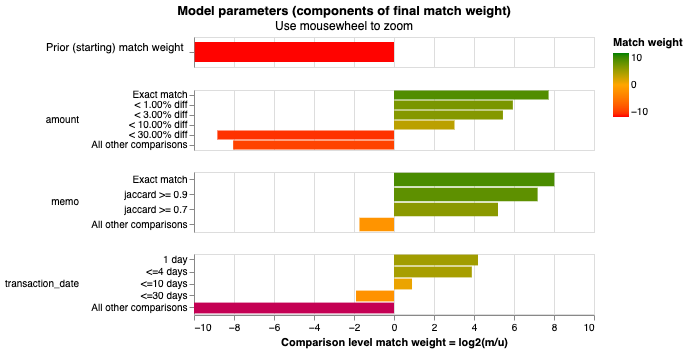df_predict = linker.predict(threshold_match_probability=0.001)

linker.comparison_viewer_dashboard(df_predict,"comparison_viewer_transactions.html", overwrite=True)
from IPython.display import IFrame
IFrame(
src="./comparison_viewer_transactions.html", width="100%", height=1200
)

pred_errors =  linker.prediction_errors_from_labels_column("ground_truth", include_false_positives=True, include_false_negatives=False)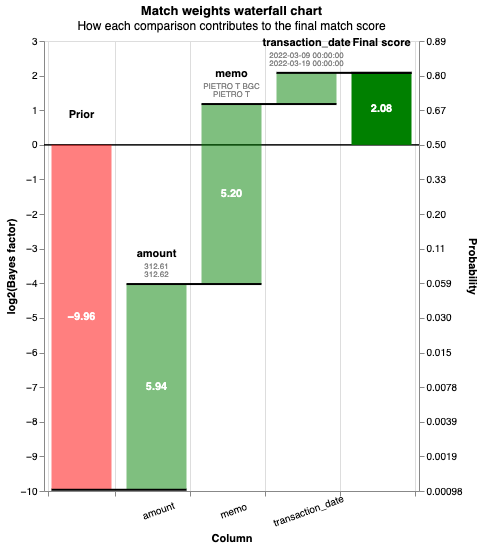pred_errors =  linker.prediction_errors_from_labels_column("ground_truth", include_false_positives=False, include_false_negatives=True)